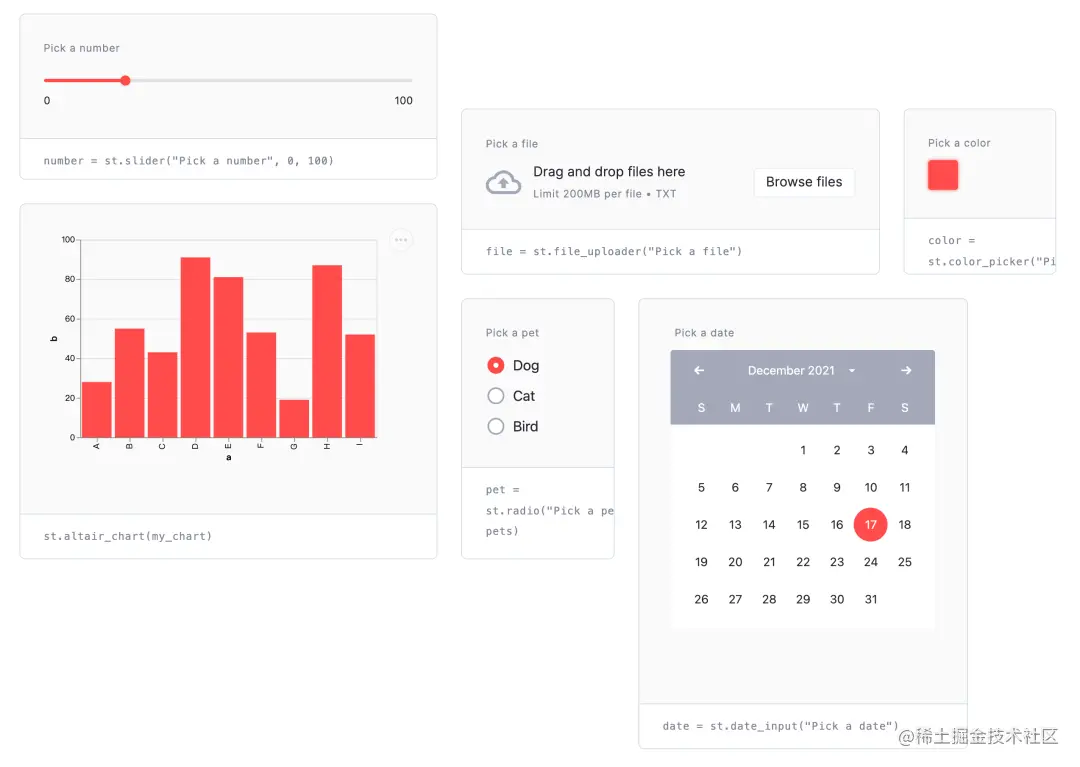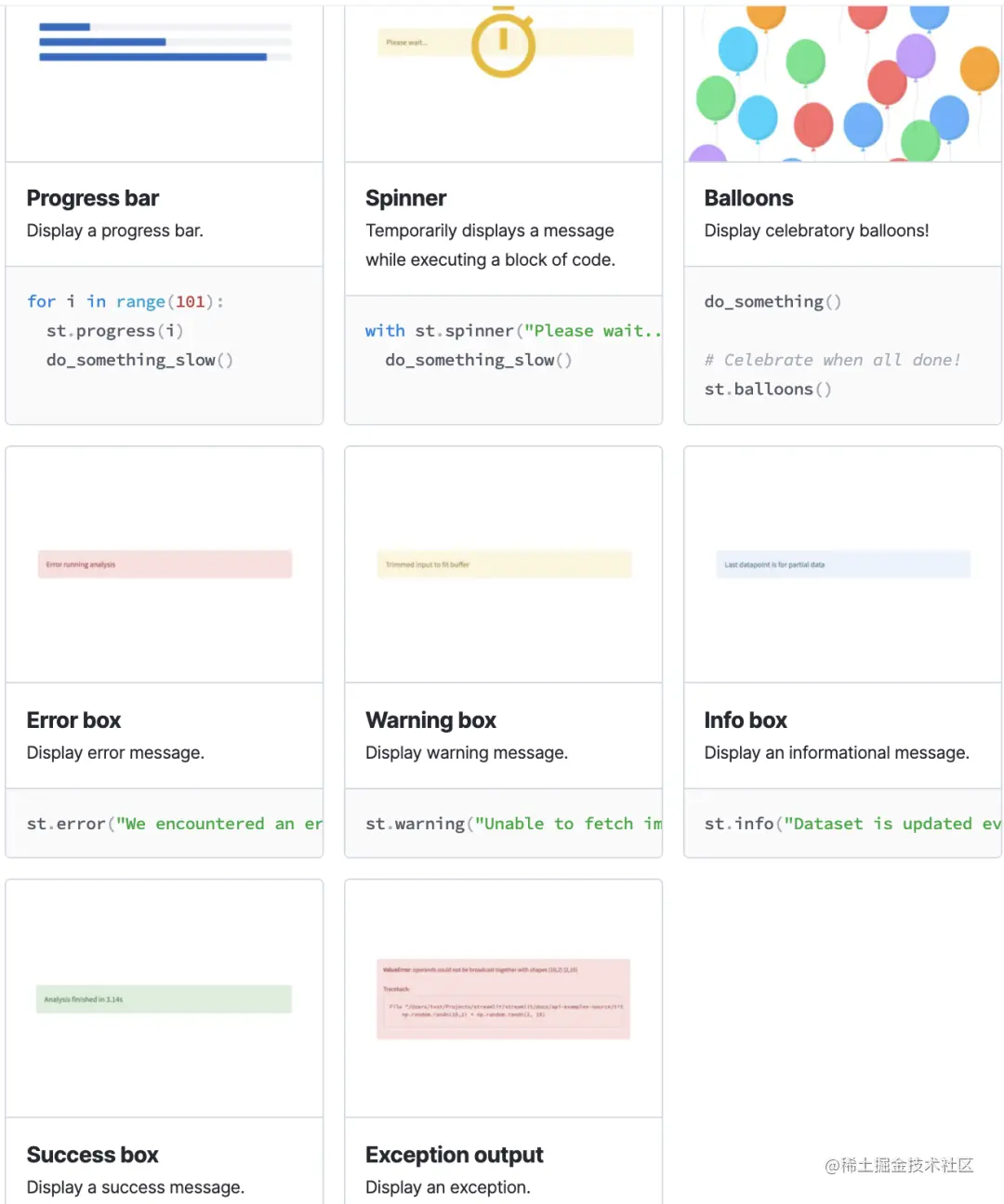# 構建可視化 web的 Python 神器streamlit

Streamlit 是一個用於機器學習、數據可視化的 Python 框架，它能幾行代碼就構建出一個精美的在線 app 應用。

## 1. 如何安裝？

`➜ pip install streamlit `

`➜ python3 -m venv .`

`➜ source ./venv/bin/activate`

```➜ pip list | wc -l
92```

## 2. 入門示例

Streamlit 提供瞭一些入門示例，執行如下命令即可

`➜ streamlit hello``➜ streamlit run st-demo.py`

## 2. Markdown 文本

• st.title()：文章大標題
• st.text()：文本
• st.code()：代碼，同時可設置代碼的語言，顯示的時候會高亮
• st.latex()：latex 公式
• st.caption()：小字體文本

```import streamlit as st

# markdown
st.markdown('Streamlit Demo')

# 設置網頁標題
st.title('一個傻瓜式構建可視化 web的 Python 神器 -- streamlit')

# 展示一級標題

st.text('和安裝其他包一樣，安裝 streamlit 非常簡單，一條命令即可')
code1 = '''pip3 install streamlit'''
st.code(code1, language='bash')

# 展示一級標題

# 展示二級標題

# 純文本
st.text('導入 streamlit 後，就可以直接使用 st.markdown() 初始化')

# 展示代碼，有高亮效果
code2 = '''import streamlit as st
st.markdown('Streamlit Demo')'''
st.code(code2, language='python')```

Streamlit 運行的方式 與普通的腳本 有所不同，應該使用 `streamlit run st-demo.py`## 3. 數據圖表支持

### 3.1 圖表組件

• table：普通的表格，用於靜態數據的展示
• dataframe：高級的表格，可以進行數據的操作，比如排序

Table 的示例：

```df = pd.DataFrame(
np.random.randn(10, 5),
columns=('第%d列' % (i+1) for i in range(5))
)

st.table(df)```Datafram 的示例：

```df = pd.DataFrame(
np.random.randn(10, 5),
columns=('第%d列' % (i+1) for i in range(5))
)

st.dataframe(df.style.highlight_max(axis=0))```• highlight_null：空值高亮
• highlight_min：最小值高亮
• highlight_max：最大值高亮
• highlight_between：某區間內的值高亮
• highlight_quantile：暫沒用過

### 3.2 監控組件

```col1, col2, col3 = st.columns(3)
col1.metric("Temperature", "70 °F", "1.2 °F")
col2.metric("Wind", "9 mph", "-8%")
col3.metric("Humidity", "86%", "4%")```### 3.3 原生圖表組件

Streamlit 原生支持多種圖表：

• st.line_chart：折線圖
• st.area_chart：面積圖
• st.bar_chart：柱狀圖
• st.map：地圖

```chart_data = pd.DataFrame(
np.random.randn(20, 3),
columns=['a', 'b', 'c'])
st.line_chart(chart_data)``````chart_data = pd.DataFrame(
np.random.randn(20, 3),
columns = ['a', 'b', 'c'])
st.area_chart(chart_data)``````chart_data = pd.DataFrame(
np.random.randn(50, 3),
columns = ["a", "b", "c"])
st.bar_chart(chart_data)``````df = pd.DataFrame(
np.random.randn(1000, 2) / [50, 50] + [37.76, -122.4],
columns=['lat', 'lon']
)
st.map(df)```### 3.4 外部圖表組件

Streamlit 的一些原生圖表組件，雖然做到瞭傻瓜式，但僅能輸入數據、高度和寬度，如果你想更漂亮的圖表，就像 matplotlib.pyplot、Altair、vega-lite、Plotly、Bokeh、PyDeck、Graphviz 那樣，streamlit 也提供瞭支持：

• st.pyplot
• st.bokeh_chart
• st.altair_chart
• st.altair_chart
• st.vega_lite_chart
• st.plotly_chart
• st.pydeck_chart
• st.graphviz_chart

## 4. 用戶操作支持

• button：按鈕
• checkbox：復選框
• selectbox：下拉單選框
• multiselect：下拉多選框
• slider：滑動條
• select_slider：選擇條
• text_input：文本輸入框
• text_area：文本展示框
• number_input：數字輸入框，支持加減按鈕
• date_input：日期選擇框
• time_input：時間選擇框
• color_picker：顏色選擇器## 5. 多媒體組件

• st.image
• st.audio
• st.video## 6. 狀態組件

• progress：進度條，如遊戲加載進度
• spinner：等待提示
• balloons：頁面底部飄氣球，表示祝賀
• error：顯示錯誤信息
• warning：顯示報警信息
• info：顯示常規信息
• success：顯示成功信息
• exception：顯示異常信息（代碼錯誤棧）## 7. 頁面佈局

Streamlit 是自上而下渲染的，組件在頁面上的排列順序與代碼的執行順序一致。

st.sidebar：側邊欄• st.columns：列容器，處在同一個 columns 內組件，按照從左至右順序展示
• st.expander：隱藏信息，點擊後可展開展示詳細內容，如：展示更多
• st.container：包含多組件的容器
• st.empty：包含單組件的容器

## 8. 流程控制系統

Streamlit 是自上而下逐步渲染出來的，若你的應用場景需要對渲染做一些控制，streamlit 也有提供對應的方法

• st.stop：可以讓 Streamlit 應用停止而不向下執行，如：驗證碼通過後，再向下運行展示後續內容。
• st.form：表單，Streamlit 在某個組件有交互後就會重新執行頁面程序，而有時候需要等一組組件都完成交互後再刷新（如：登錄填用戶名和密碼），這時候就需要將這些組件添加到 form 中
• st.form_submit_button：在 form 中使用，提交表單。

## 9. 緩存特性提升速度

```DATE_COLUMN = 'date/time'
DATA_URL = ('https://s3-us-west-2.amazonaws.com/'
'streamlit-demo-data/uber-raw-data-sep14.csv.gz')

@st.cache
lowercase = lambda x: str(x).lower()
data.rename(lowercase, axis='columns', inplace=True)
data[DATE_COLUMN] = pd.to_datetime(data[DATE_COLUMN])
return data```

## 10. 部署上線• requirements.txt：依賴包文件
• setup.sh：安裝腳本，主要是創建文件夾，寫入配置文件
• Procfile：啟動腳本，告訴 Heroku 如何安裝並啟動應用```git init
git commit -m "init"```

```heroku login
heroku create
git push heroku master
heroku ps:scale web=1```

`heroku logs --tail`

`heroku domains:add hivecnstats.iswbm.com`

## 12. 總結

Streamlit 一個開箱即用的工具集，它可以讓一個普通的個人開發者免於學習繁雜的前端知識，就可以輕松、快速的構建一個簡潔、優雅的 web app 應用，這是 streamlit 最吸引人的地方。